### Day 66 - Differentiation using the Natural Logarithmic Function - 04.22.15

Update
• Unit 5 Test, next Friday!

Questions

Bell Ringer
1. Differentiation of ln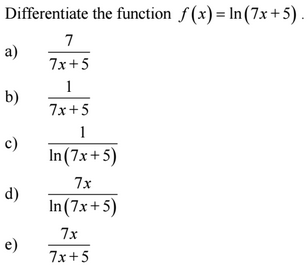1. Differentiation of ln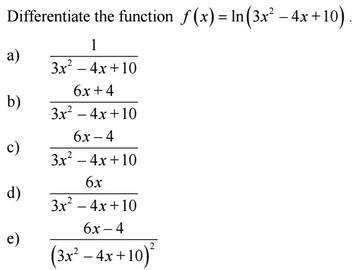1. Differentiation of ln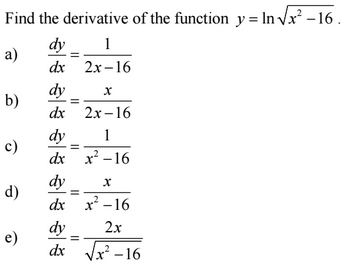1. Differentiation of ln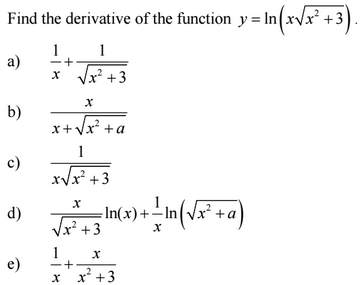1. Differentiation of ln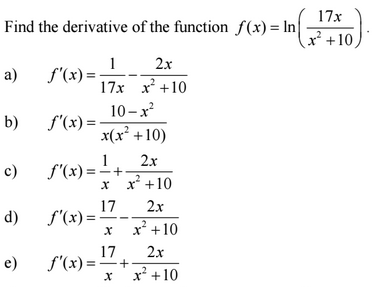Review
• Prerequisites
• Derivatives
• Derivative Rules
• Power Rule
• Constant Rule
• Trig Derivatives
• Logarithm Properties

Lesson
• Differentiation of Logarithmic Functions (video)

Exit Ticket
• Posted on the board at the end of the block!

Lesson Objective(s)
• How can the derivative of natural logarithmic functions be calculated?
Skills
1. Explain why the power rule does not work when integrating 1/x.
2. Calculate the derivative of the natural logarithmic function.

#### In-Class Help Requests

Standard(s)
• APC.9
• Apply formulas to find derivatives.
• Includes:
• derivatives of algebraic, trigonometric, exponential, logarithmic, and inverse trigonometric functions
• derivations of sums, products, quotients, inverses, and composites (chain rule) of elementary functions
• derivatives of implicitly defined functions
• higher order derivatives of algebraic, trigonometric, exponential, and logarithmic, functions